Next: CM-5 Communications Model Up: Parallel Algorithm Analysis Previous: Parallel Algorithm Analysis

## Parallel Communications Model

The model we present assumes that processors pass messages to each other via a communications network. In this model, a processor sending a message incurs overhead costs for startup such as preparing a message for transmission, alerting the destination processor that it will receive this message, and handling the destination's acknowledgment. We call this time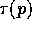.

The sending processor partitions the outgoing message into sections of length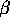bits, determined by hardware constraints. Each packet, with routing information prepended and an integrity check field using a cyclic redundancy code appended, is sent through the data network. The destination processor then reassembles the complete message from the received packets.

The communications time to send a message with this model is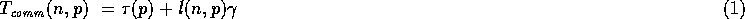where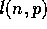is the message length, and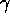is the transmission rate of the source into the network measured in seconds per packet.

Fortunately, all the algorithms described in this paper use regular communication patterns whose complexities can be easily estimated on the two architectures of the CM-2 and of the CM-5. Each such communication pattern can be expressed as a constant number of block permutations, where given blocks of contiguous data,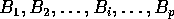, residing in processors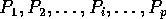, and a permutation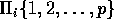, block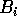has to be sent from processor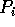to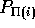, where each block is of the same size, say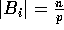. As we illustrate next, on both the CM-2 and the CM-5, the communication complexity of a regular block permutation can be expressed as follows: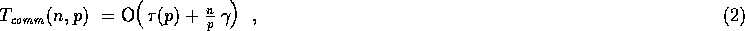where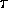is the start-up cost andis the packet transmission rate. We next address how to estimate the communication complexity for operations on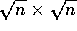images and their relationship to block permutations.Next: CM-5 Communications Model Up: Parallel Algorithm Analysis Previous: Parallel Algorithm Analysis